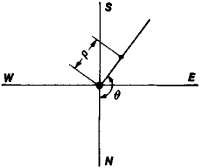# position micrometer

Also found in: Dictionary, Wikipedia.

## position micrometer

See filar micrometer.

## Position Micrometer

an instrument for jointly determining small distances ρ and position angles θ in the focal plane of an optical instrument (Figure 1). It is usually in the form of a cross-wire micrometer equipped with a divided circle. AFigure 1. Position angle β and distance ρ

position micrometer can be used to measure distances at various angles to an hour circle (position angles); the angles are read from the divided circle. It is also used to measure the relative positions of various celestial objects, particularly the orbital parameters of binary stars and planetary satellites.

Site: Follow: Share:
Open / Close